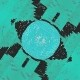# Semicircle Turducken

Here’s another of those Catriona Shearer puzzles that had me stumped for a while.

7.
Semicircle TurduckenThis looks ridiculous. There are no lengths, areas, or angles given, but you’re supposed to figure out an angle?

Spoiler!

I added some more lines and labeled some points.(I deliberately made the triangle more asymmetric.)

BC is a diameter of the light blue (semi)circle and A lies on that (semi)circle. That means angle CAB is a right angle, and angles CBA and ACB are complementary.

The dark blue circle is inscribed in triangle ABC. The contact points are D, E, and F, and the triangles EAF, FBD, and DCE are isosceles.

The center of the inscribed circle, O, is the mutual intersection point of the bisectors of angles ABC, BCA, and CAB. Since, for instance, BO bisects angle ABC, that means BO bisects line segment DF at the point H, and BHD is a right angle. Likewise BDO, being the angle between a radius and a tangent of the circle, is a right angle.

So then triangles HOD and ODB are similar, and angle ODH is congruent to angle DBO, which is half of angle CBA.

Similarly, angle ODJ is half of angle ACB.

And that means HDJ, the requested angle, is half the sum of complementary angles CBA and ACB: 45°.

## 2 thoughts on “Semicircle Turducken”

1.sansdomino says:

Maybe more simple: note that angles BAC = FAE, AFO and AEO are each 90°, and therefore angle FOE is as well; then apply the inscribed angle theorem.

2.Richard Holmes says:

Sure, that works, if you remember the inscribed angle theorem!